Documentation

### This is machine translation

Mouseover text to see original. Click the button below to return to the English version of the page.

To view all translated materials including this page, select Country from the country navigator on the bottom of this page.

## Modeling Mutual Coupling in Large Arrays Using Embedded Element Pattern

The pattern multiplication principle states that the radiation pattern of an array can be considered as the multiplication of the element pattern and the array factor. However, when an antenna gets deployed into an array, its radiation pattern is modified by its neighboring elements. This effect is often referred to as mutual coupling. Thus, to improve the fidelity of the analysis, one should use the element pattern with mutual coupling effect in the pattern multiplication instead of the isolated element (an element located in space by itself) pattern.

Unfortunately it is often very difficult to model the exact mutual coupling effect among elements. This example shows one possible approach to model the mutual coupling effects via an embedded pattern, which refers to the pattern of a single element embedded in a finite array. The element of choice is in general at the center of the array. The embedded pattern is calculated or measured by transmitting through the element itself while terminating all other elements in the array with a reference impedance -. This approach works well when the array is large so the edge effects may be ignored.

The example models two arrays: first using the pattern of the isolated element, second with the embedded element pattern and compare the results of the two with the full-wave Method of Moments (MoM) based solution of the array. The array performance for scanning at broadside, and for scanning off broadside is established. Finally, the array spacing is adjusted to investigate the occurrence of scan blindness and compare against reference results .

This example requires Antenna Toolbox™.

### Model an Array of Dipoles Using Isolated Element Pattern

First, we design an array with isolated element. For this example we choose the center of the X-band as our design frequency.

```freq = 10e9; vp = physconst('lightspeed'); lambda = vp/freq; ```

In , it was discussed that the central element of a 5X 5array, whereis the wavelength, starts to behave like it is in an infinite array. Such an aperture would correspond to a 10 X 10 array of half-wavelength spaced radiators. We choose to slightly exceed this limit and consider a 11 X 11 array of dipoles.

```Nrow = 11; Ncol = 11; drow = 0.5*lambda; dcol = 0.5*lambda; ```

The dipole of choice has a length slightly lower thanand a radius of approximately.

```mydipole = dipole; mydipole.Length = 0.47*lambda; mydipole.Width = cylinder2strip(0.191e-3); figure('Color','w'); show(mydipole); ```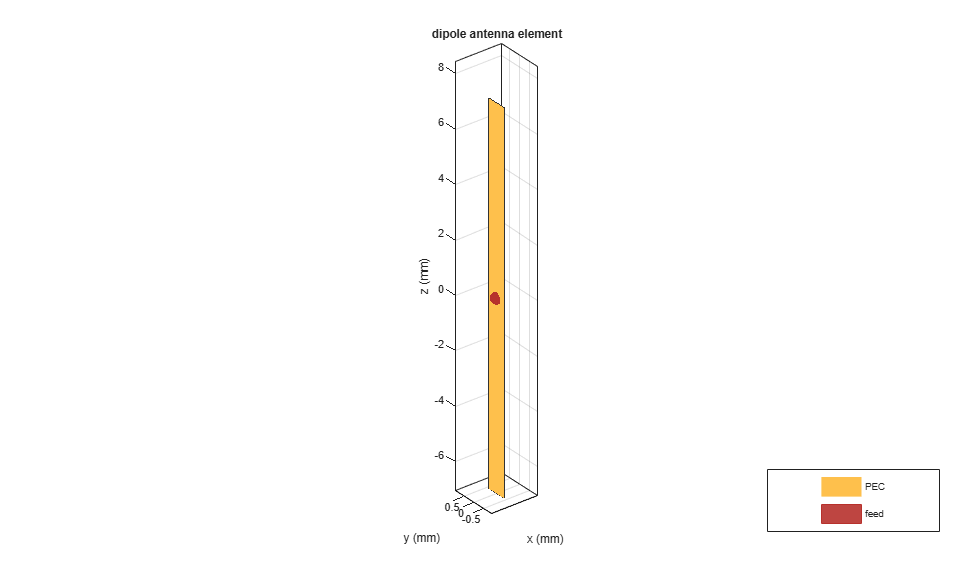Now creates an 11 X 11 URA and assign the isolated dipole as its element. Adjust the element spacing to be half-wavelength at 10 GHz. The dipole tilt is set to zero so its orientation matches the array geometry in the Y-Z plane.

```isolatedURA = phased.URA; isolatedURA.Element = mydipole; isolatedURA.Size = [Nrow Ncol]; isolatedURA.ElementSpacing = [drow dcol]; viewArray(isolatedURA); myFigure = gcf; myFigure.Color = 'w'; ```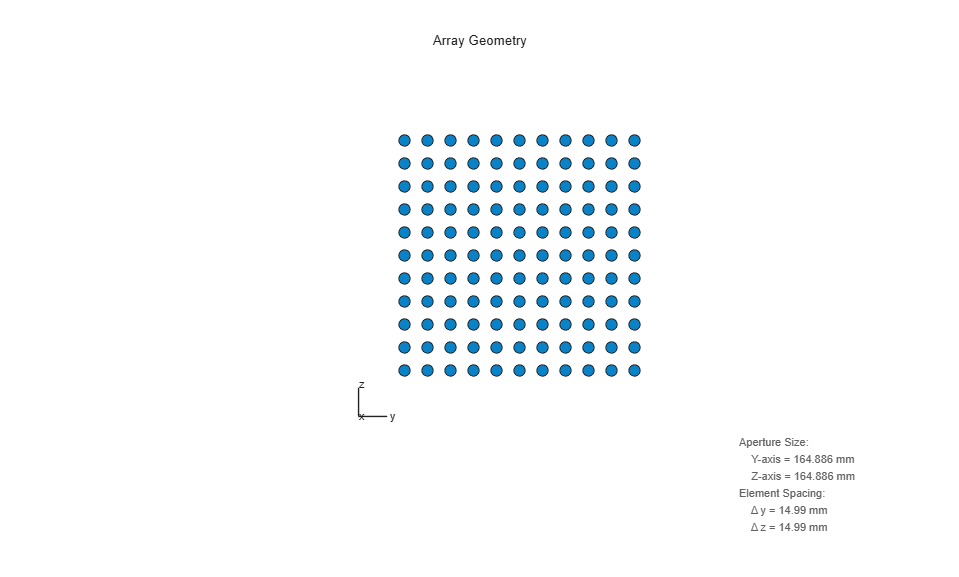### Model Array of Dipoles Using Embedded Element Pattern

To compute the embedded pattern of the center dipole element, we first create a full-wave model of the previous array. Since the default orientation of the dipole element in the library is along z-axis, we tilt it so that the array is formed in the X-Y plane.

```fullWaveArray = rectangularArray(... 'Size',[Nrow Ncol],... 'RowSpacing',drow,... 'ColumnSpacing',dcol); fullWaveArray.Element = mydipole; fullWaveArray.Element.Tilt = 90; fullWaveArray.Element.TiltAxis = [0 1 0]; show(fullWaveArray) title('Rectangular 11 X 11 Array of Dipole Antennas') ```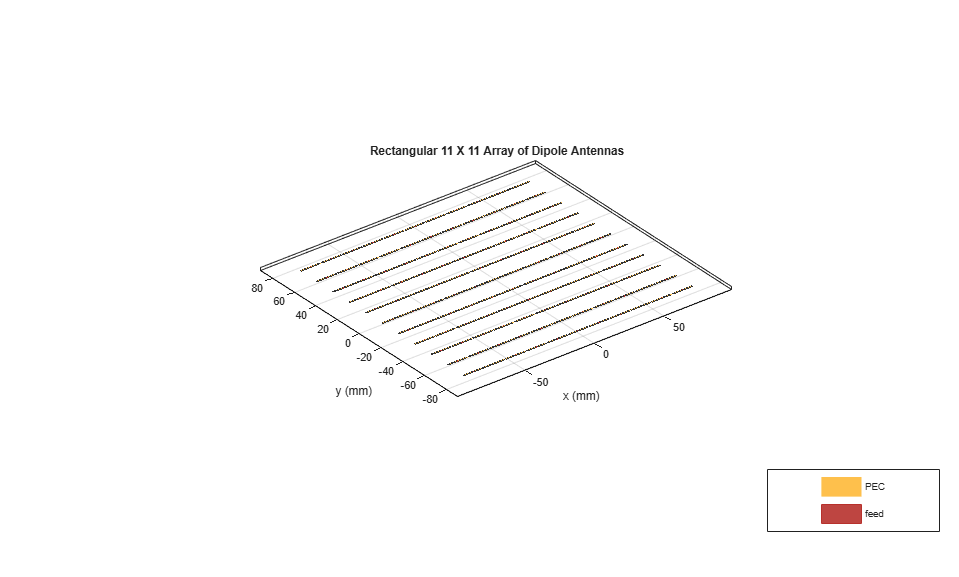To calculate the embedded element pattern, use the `pattern` function and pass in additional input parameters of the element number(index of the center element) and termination resistance. The scan resistance and scan reactance for an infinite array of resonant dipoles spacedapart is provided in  and we choose the resistance at broadside as the termination for all elements.

```Zinf = 76 + 1i*31; ElemCenter = (prod(fullWaveArray.Size)-1)/2 + 1; az = -180:2:180; el = -90:2:90; EmbElFieldPatCenter = pattern(fullWaveArray,freq,az,el,... 'ElementNumber',ElemCenter,'Termination',real(Zinf),'Type','efield'); ```

Import this embedded element pattern into a custom antenna element and create the same rectangular array using that element. Since the array will be in the Y-Z plane, rotate the pattern to match the scan planes.

```embpattern = helperRotatePattern(az,el,EmbElFieldPatCenter,[0 1 0],90); embpattern = mag2db(embpattern); fmin = freq - 0.1*freq; fmax = freq + 0.1*freq; freqVector = [fmin fmax]; embantenna = phased.CustomAntennaElement('FrequencyVector',freqVector,... 'AzimuthAngles',az,'ElevationAngles',el,... 'MagnitudePattern',embpattern,'PhasePattern',zeros(size(embpattern))); embeddedURA = phased.URA; embeddedURA.Element = embantenna; embeddedURA.Size = [Nrow Ncol]; embeddedURA.ElementSpacing = [drow dcol]; ```

### Compare Array Pattern in Elevation and Azimuth Plane

Next, calculate and compare the patterns in different planes for the three arrays : the one using the isolated element pattern, the one using the embedded element pattern, and the full-wave model (used as the ground truth).

First, the pattern in the elevation plane (specified by azimuth = 0 deg and also called the E-plane)

```Eplane_embedded = pattern(embeddedURA,freq,0,el); Eplane_isolated = pattern(isolatedURA,freq,0,el); [Eplane_fullwave,~,el3e] = pattern(fullWaveArray,freq,0,0:1:180); el3e = el3e'-90; helperATXPatternCompare([el(:) el(:) el3e(1:2:end)],... [Eplane_isolated Eplane_embedded Eplane_fullwave(1:2:end)],... 'Elevation Angle (deg.)','Directivity (dBi)',... 'E-plane Array Directivity Comparison',... {'With Isolated Pattern','With Embedded Pattern',... 'Full Wave Solution'},[-60 30]); ```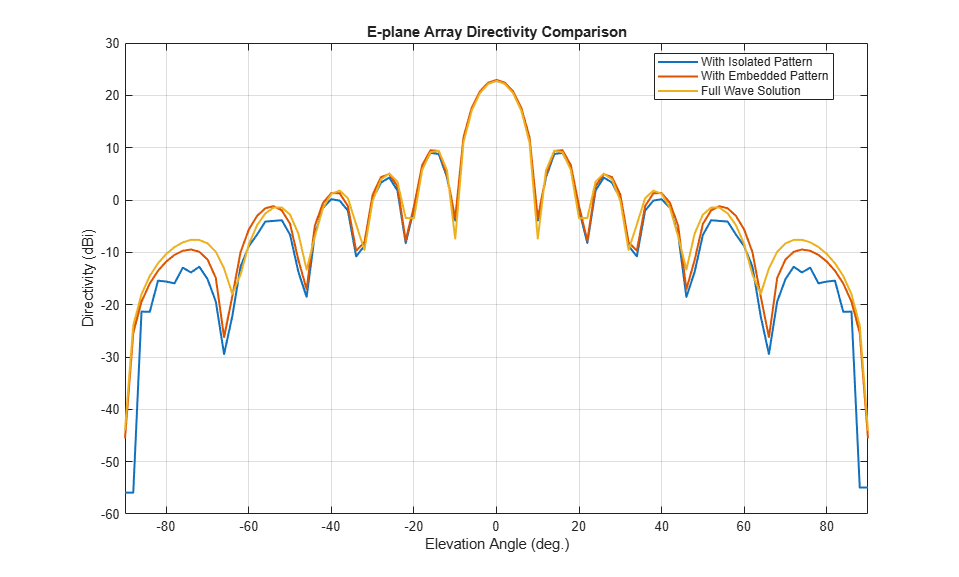Now, the pattern in the azimuth plane (specified by elevation = 0 deg and called the H-plane).

```Hplane_embedded = pattern(embeddedURA,freq,az/2,0); Hplane_isolated = pattern(isolatedURA,freq,az/2,0); Hplane_fullwave = pattern(fullWaveArray,freq,90,0:1:180); helperATXPatternCompare([az(:)/2 az(:)/2 el3e],... [Hplane_isolated Hplane_embedded Hplane_fullwave],... 'Azimuth Angle (deg.)','Directivity (dBi)',... 'H-plane Array Directivity Comparison',... {'With Isolated Pattern','With Embedded Pattern',... 'Full Wave Solution'},[-60 30]); ```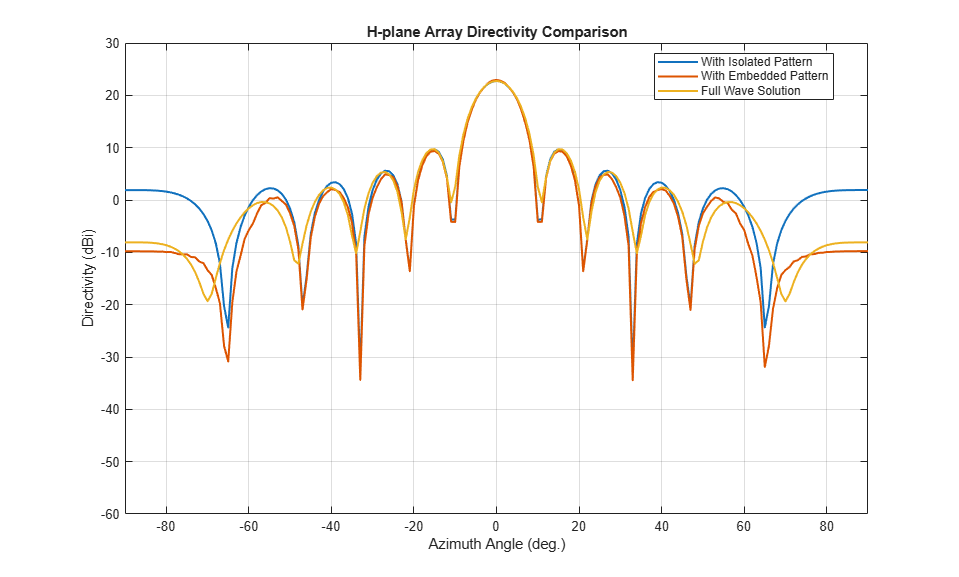The array directivity is approximately 23 dBi. This result is close to the theoretical calculation for the peak directivity  after taking into account the absence of a reflector, D = 4/,.

The pattern comparison suggests that the main beam and the first sidelobes are aligned for all three cases. Moving away from the main beam shows the increasing effect of coupling on the sidelobe level. As expected,the embedded element pattern approach suggests a coupling level in between the full-wave simulation model and the isolated element pattern approach.

### Increase Array Size

The behavior of the array pattern is intimately linked to the embedded element pattern. To understand how our choice of a 11 X 11 array impacts the center element behavior, we increase the array size to a 25 X 25 array (12.5X 12.5aperture size). Note that the triangular mesh size for the full wave Method of Moments (MoM) analysis with 625 elements increases to 25000 triangles (40 triangles per dipole) and the computation for the embedded element pattern takes approximately 12 minutes on a 2.4 GHz machine with 32 GB memory. This time can be reduced by lowering the mesh size per element by meshing manually using a maximum edge length of.

Below is the pattern plot for the E-plane,

```load atexdipolearray embpattern = helperRotatePattern(... DipoleArrayPatData.AzAngles,DipoleArrayPatData.ElAngles,... DipoleArrayPatData.ElemPat(:,:,3),[0 1 0],90); embpattern = mag2db(embpattern); embantenna2 = clone(embantenna); embantenna2.AzimuthAngles = DipoleArrayPatData.AzAngles; embantenna2.ElevationAngles = DipoleArrayPatData.ElAngles; embantenna2.MagnitudePattern = embpattern; embantenna2.PhasePattern = zeros(size(embpattern)); Eplane_embedded = pattern(embantenna2,freq,0,el); Eplane_embedded = Eplane_embedded - max(Eplane_embedded); % normalize Eplane_isolated = pattern(mydipole,freq,0,el); Eplane_isolated = Eplane_isolated - max(Eplane_isolated); % normalize embpatE = pattern(embantenna,freq,0,el); embpatE = embpatE-max(embpatE); % normalize helperATXPatternCompare([el(:) el(:) el(:)],... [Eplane_isolated embpatE Eplane_embedded],... 'Elevation Angle (deg.)','Directivity (dBi)',... 'Normalized E-plane Element Directivity Comparison',... {'Isolated Pattern','Embedded Pattern - 11 X 11',... 'Embedded Pattern - 25 X 25'},[-50 5]); ```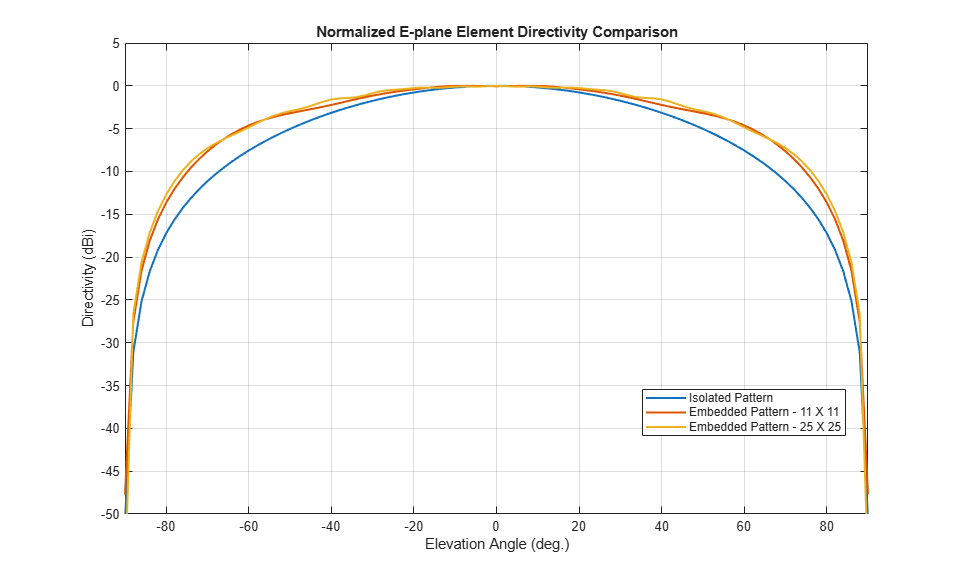and the H-plane.

```Hplane_embedded = pattern(embantenna2,freq,0,az/2); Hplane_embedded = Hplane_embedded - max(Hplane_embedded); % normalize Hplane_isolated = pattern(mydipole,freq,0,az/2); Hplane_isolated = Hplane_isolated - max(Hplane_isolated); % normalize embpatH = pattern(embantenna,freq,az/2,0); embpatH = embpatH-max(embpatH); % normalize helperATXPatternCompare([az(:)/2 az(:)/2 az(:)/2],... [Hplane_isolated embpatH Hplane_embedded],... 'Azimuth Angle (deg.)','Directivity (dBi)',... 'Normalized H-plane Element Directivity Comparison',... {'Isolated Pattern','Embedded Pattern - 11 X 11',... 'Embedded Pattern - 25 X 25'},[-50 5]); ```The plot above reveals that the difference between embedded element patterns of the 11 X 11 and the 25 X 25 array, respectively, is less than 0.5 dB, in the E-plane. However, the H-plane shows more variation for the 11 X 11 array as compared with the 25 X 25 array.

### Scan Behavior and Embedded Element Pattern

This section scans the array based on the embedded element pattern in the elevation plane defined by azimuth = 0 deg and plot the normalized directivity. In addition, the normalized embedded element pattern is also plotted. Note the overall shape of the normalized array pattern approximately follows the normalized embedded element pattern, just as predicted by the pattern multiplication principle.

```eplane_indx = find(az==0); scan_el1 = -30:10:30; scan_az1 = zeros(1,numel(scan_el1)); scanEplane = [scan_az1;scan_el1]; % compute array scanning weights steeringvec = phased.SteeringVector('SensorArray',embeddedURA,... 'IncludeElementResponse',true); weights = steeringvec(freq,scanEplane); % array scanning legend_string1 = cell(1,numel(scan_el1)); scanEPat = nan(numel(el),numel(scan_el1)); for i = 1:numel(scan_el1) scanEPat(:,i) = pattern(embeddedURA,freq,scan_az1(i),el,... 'Weights',weights(:,i)); legend_string1{i} = strcat('scan = ',num2str(scan_el1(i))); end scanEPat = scanEPat - max(max(scanEPat)); % normalize helperATXPatternCompare(el(:),scanEPat,... 'Elevation Angle (deg.)','Directivity (dBi)',... 'E-plane Scan Comparison',legend_string1(1:end-1),[-50 5]); hold on; plot(el(:),embpatE,'-.','LineWidth',1.5); legend([legend_string1,{'Embedded element'}],'location','best') hold off; ```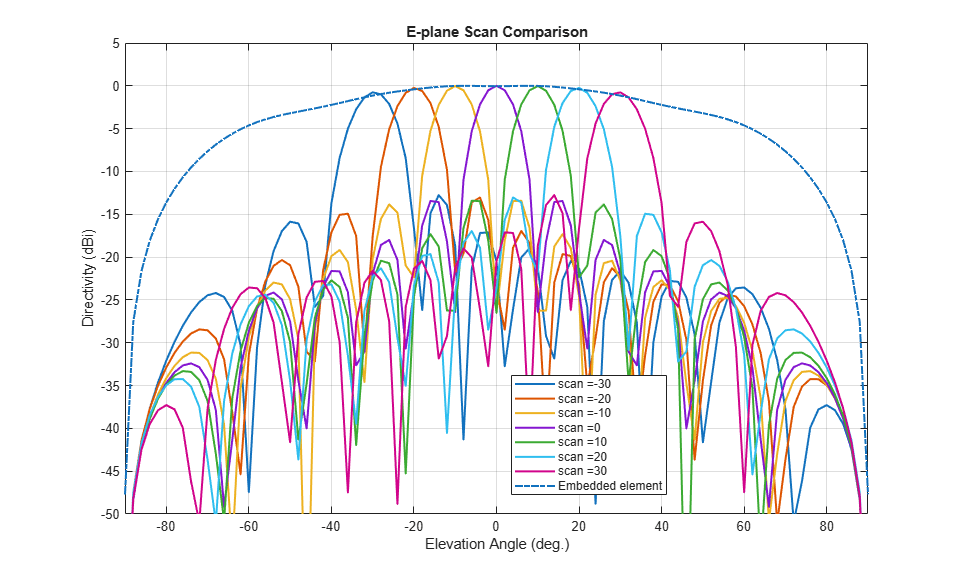Scan Blindness

In large arrays, the array directivity can reduce drastically at certain scan angles under certain situations. At these scan angles, referred to as the blind angles, the array does not radiate the power supplied at its input terminals . Two common mechanisms under which blindness conditions occur are

• Surface Wave Excitation

• Grating Lobe Excitation

It is possible to detect scan blindness in large finite arrays by studying the embedded element pattern (also known as array element pattern in the infinite array analysis). The array being investigated in this example does not have a dielectric substrate/ground plane, and therefore the surface waves are eliminated. However we can investigate the second mechanism, i.e. the grating lobe excitation. To do so, let us increase the spacing across rows and columns of the array to be 0.7. Since this spacing is greater than the half-wavelength limit we should expect grating lobes in the visible space beyond a specific scan angle. As pointed out in , to accurately predict the depth of grating lobe blind angles in the finite array of dipoles, we need to have an array of the size 41 X 41 or higher. We will compare 3 cases, namely the 11 X 11, 25 X 25 and the 41 X 41 size arrays and check if the existence of blind angles can at least be observed in the 11 X 11 array. As mentioned earlier, the results were precomputed in Antenna Toolbox™ and saved in a MAT file. To reduce the computational time, the elements were meshed with maximum edge length of.

```load atexdipolearrayblindness.mat ```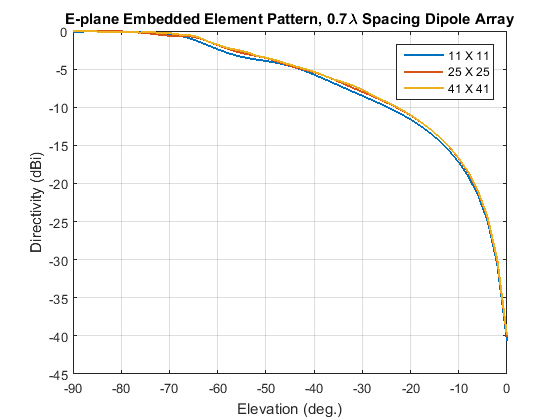The normalized E-plane embedded element pattern for arrays of three sizes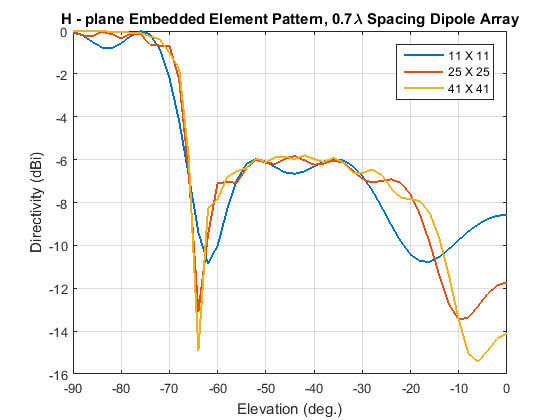The normalized H-plane embedded element pattern for arrays of three sizes. Notice the blind angle around -62 and -64 deg.

### Conclusion

The embedded element pattern approach is one possible way to perform the analysis of large finite arrays. They need to be large enough so that the edge effects can be ignored. The approach replaces the isolated element pattern with the embedded element pattern since the latter includes the effect of mutual coupling.

### Reference

 R. J. Mailloux, 'Phased Array Antenna Handbook', Artech House,2nd edition, 2005

 W. Stutzman, G. Thiele, 'Antenna Theory and Design', John Wiley & Sons Inc., 3rd Edition, 2013.

 R. C. Hansen, Phased Array Antennas, Chapter 7 and 8, John Wiley & Sons Inc.,2nd Edition, 1998.

 H. Holter, H. Steyskal, "On the size requirement for finite phased-array models," IEEE Transactions on Antennas and Propagation, vol.50, no.6, pp.836-840, Jun 2002.

 P. W. Hannan, "The Element-Gain Paradox for a Phased-Array Antenna," IEEE Transactions on Antennas Propagation, vol. 12, no. 4, July 1964, pp. 423-433.